# Trying some physics i don't even know about.

Sometimes I get so bored that I just try to calculate certain experiments I've heard without know the solution. Like using the basic formula to calculate the speed of the moon around the Earth. This time I tried to calculate the differences between 2 clocks, on put on the highest building in the world (Burj Khalifa) with 828m of the ground and one in its basement so 0m.

First of all, I went searching for some variables I need to use :
• Rotation of the Earth = 23h 59min 04s = 86164 s
• Radius of Earth = 6378 x 103 m
• Speed of light = 299 792 458 m/s

I also found the formula I needed to use :To find the Velocity, I used the simple formulaandSo, I'm trying to find the velocity of those 2 clocks relative to the center of the earth. And because one clock is further (as we see in the formula, if the angular speed is constant) it would have a faster velocity so it should go faster in time.

Velocity clock basement :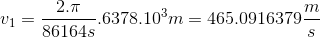Velocity clock on top :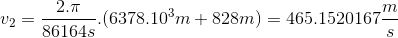Then I chose for a timeframe of an hour = 3600s

That means I need to calculate the space they have done with their velocity :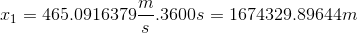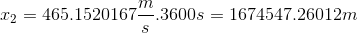Now we should have all the variables for the formula I first mentioned, so let's fill it in :D

For the clock in the basement :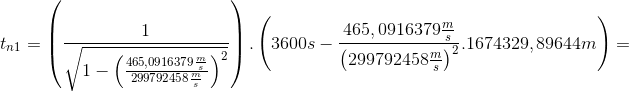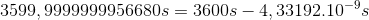So 4.33192 nanoseconds faster than the center of the earth.

Now for the clock on top :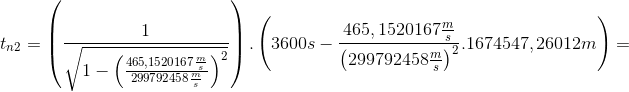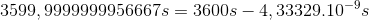So 4.33329 nanoseconds faster than the center of the earth.

There seems to be a theoretical a difference of 1.37 picoseconds after 1h on the clocks.

First of all, this is my first time trying to solve such a question, I just graduated high school. But I'm really interested in this field of physics.

If I made a mistake somewhere I would love to hear it, also English grammar mistakes will be visible, my apologize for those also.

Have a great day further.

#### Attachments

PeroK
Homework Helper
Gold Member
2021 Award
I also found the formula I needed to use :The first formula relates the time of an event in two reference frames related by the Lorentz Transformation for a relative velocity ##v## in the x-direction, and isn't relevant here.

The second formula, for the gamma factor, is relevant here.

Note you you may also want to take gravitational time dilation into account. See:

https://en.wikipedia.org/wiki/Gravitational_time_dilation

Note also that "moving clocks run slow" in your reference frame.

You may also be interested in the famous Hafele-Keating experiment:

https://en.wikipedia.org/wiki/Hafele–Keating_experiment

Oh well, I've still got a lot to learn, but thx for the referrals, I'll try to understand them :)

PeroK